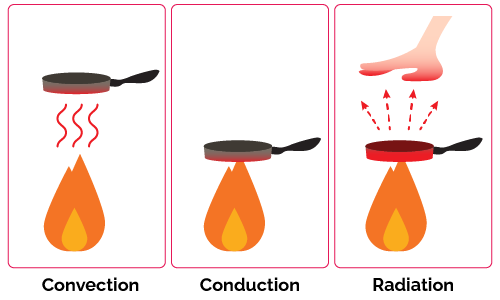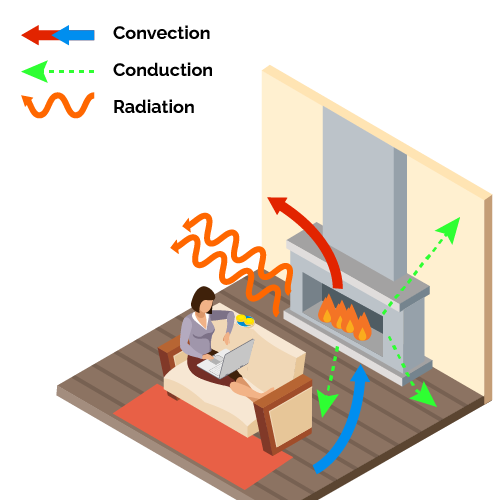# Heat Transfer Calculator

Created by Joanna Śmietańska
Last updated: Jan 20, 2023

With this heat transfer calculator, finding the heat rates for different types of heat transfer will be no challenge for you! Heat transfer occurs every time you bring objects of different temperatures into contact with each other. So, there are many examples of heat transfer in daily life when heat is transferred from one object to another.

The concept of heat and the mechanisms of heat transfer are central to engineering and industrial and domestic equipment design. In the text below, we will discuss the heat transfer formula. So let's start by explaining what heat transfer is!

## Heat transfer definition – types of heat transfer

Heat transfer occurs when one system comes into contact with another low-temperature system. The energy in the form of heat is transferred from the molecules in the first system to the second system. When the temperature increases, the kinetic energy of the molecules also increases. We explained more about heat flow in our thermal equilibrium calculator.

There are three different types of heat transfer:

• Conductive heat transfer – the transfer of heat from one molecule to another through direct contact between objects. Conduction is a very effective method of heat transfer in metals, but gases such as air conduct heat poorly.

• Convective heat transfer – usually refers to fluids or gases that exchange heat with other objects during their free movement. See how a fireplace works: heated air expands and rises throughout the room, while cooler air descends towards the fire where it is heated.

• Radiative heat transfer – electromagnetic waves can also transfer heat when they come into contact with matter. The more radiation a body absorbs, the higher the heat transfer. As an example of heat transfer, white objects absorb very little heat – check that they will be slower to heat up during hot summers.## Heat transfer equations

This heat transfer calculator can match the formula depending on which type of heat transfer you are dealing with. The basic formula for the amount of heat transferred from one object to another is as follows:

$Q = m \cdot c \cdot \Delta T$

where:

• $Q$Heat transferred;
• $m$Mass of a system;
• $c$Specific heat, defined as the amount of heat required to increase the temperature of 1 kg of mass by 1 °C; and
• $\Delta T$ – Temperature difference, $\Delta T = T_2 - T_1$.

Note that heat transfer can be either positive (transfer to a system) or negative (transfer from a system).

The heat transfer rate through a slab of material depends on the type of material, the temperature difference $\Delta T$ between hot and cold sides, and the path the heat travels. All these quantities, which can be deduced from the experiment, are bound by the conduction heat transfer formula:

$Q = \frac{k \cdot A \cdot t \cdot \Delta T}{l}$

where:

• $Q$Conduction heat transfer;
• $k$Thermal conductivity of the material, visit our thermal conductivity calculator to calculate it on your own;
• $A$Surface area;
• $t$Time needed for heat transfer;
• $\Delta T$ - Temperature difference, $\Delta T = T_h - T_c$, and
• $l$Thickness of the material.

💡 In developing insulation, look at the $l/k$ ratio in the equation. The lower the conductivity $k$ and the greater the thickness $l$, the better the insulator. Check our insulation calculator to explore this topic.

Next, let's consider a material with surface area $A$, convective heat transfer coefficient $H_c$, and temperature difference $\Delta T$ between the surface and the bulk fluid. Our heat transfer calculator uses following formula for convective heat transfer:

$Q = H_c \cdot A \cdot \Delta T$

Note that the heat transfer unit per time is watts.

Finally, the radiative heat transfer formula for an object at temperature $T_1$ surrounded by an environment at temperature $T_2$ is:

$Q = \sigma \cdot e \cdot A \cdot (T_2^{4}-T_1^{4})$

where:

• $\sigma$Stefan-Boltzmann constant equal to 5.67×10−8 J/(s·m2·k4); and
• $e$emissivity of the object, it ranges from 0 (perfect reflector) to 1 (black body).

If heat transfer occurs only via emission, our Stefan Boltzmann law calculator will be perfect for you.

## Heat transfer examples in everyday life

Heat transport is critical and can be realized through conduction, convection, and radiation. We see many examples in our daily lives:

Conduction:

• Touching a hot pan and being burned.
• Ice melts in your hand.
• Holding a hot cup of coffee.

Convection:

• Barista “steams” cold milk to make hot coffee.

• Heat from the sun warming your skin.
• Heat from a lightbulb.
• Heat from a fire.
• Heating food in a microwave.

Of course, heat transfer can take place in several ways simultaneously. For example, heat is transferred in a fireplace by radiation, convection of cold air into the room and hot air into the chimney, and conduction through the floor and walls.## How to use heat transfer calculator?

This heat transfer calculator will help you compute rates for each type of heat transfer. To do it:

1. Choose a type of heat transfer, e.g. we want to calculate fluid convection.

2. Enter a heat transfer coefficient. Let's assume it is 2000 W/m2·K.

3. Specify the surface area of 1 m2.

4. Enter the bulk temperature of 20 °C and surface temperature of 50 °C.

5. Well done! Your convective heat transfer is 60,000 W or 60 kW.

## FAQ

### What is heat transfer?

Heat transfer is a process in which the thermal energy of molecules is moved from the region of a higher temperature to a lower temperature. The heat transfer unit is usually the joule, or in the case of heat transfer per unit time, it is watts or kilocalories per second.

### What are the three types of heat transfer?

The three types of heat transfer are conduction, convection, and radiation. Conduction is the transfer of energy from one molecule to another by direct contact. Convection is the movement of heat by a fluid, such as water or air. Radiation is the transfer of heat by electromagnetic waves.

### Which method of heat transfer can occur in empty space?

Only radiation heat transfer can occur in empty space. In this case, the heat transfer process does not depend on contact between the heat source and the material to be heated. Both conduction and convection need a medium to transfer heat.

### What is the radiative heat transfer from the warm object?

If an object has an area of 1 m2, an emissivity of 0.67, a temperature of 100 °C, and an environment of 0 °C, then heat transfer will be -525.09 W.

1. Use the formula: Q = σeA(T24-T14).

2. Convert temperature to kelvins: K = °C + 273.15 K.

3. Calculate heat transfer:

Q = 5.670367×10−8 × 0.67 × 1 m2 × [(373.15 K)4 - (273.15 K)4] = -525.09 W.

Mark the negative sign – the object radiates heat from the system.

Joanna Śmietańska
I want to calculate
Basic heat transfer
Data required
Mass (M)
kg
Specific heat (C)
J/(kg·K)
Initial temperature (T1)
°C
Final temperature (T2)
°C
Result
Heat transfer (Q)
J
People also viewed…

### Current divider

Use the current divider calculator to estimate how the current divides across each branch of different parallel circuits: resistive, inductive, or capacitive.

### Density to weight

The density to weight calculator goes from the density of a substance to its weight, given you know its volume.

### Discount

Discount calculator uses a product's original price and discount percentage to find the final price and the amount you save.